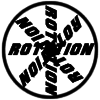#### You may also like### 8 Methods for Three by One

This problem in geometry has been solved in no less than EIGHT ways by a pair of students. How would you solve it? How many of their solutions can you follow? How are they the same or different? Which do you like best?### Rots and Refs

Follow hints using a little coordinate geometry, plane geometry and trig to see how matrices are used to work on transformations of the plane.### Reflect Again

Follow hints to investigate the matrix which gives a reflection of the plane in the line y=tanx. Show that the combination of two reflections in intersecting lines is a rotation.

# Square Pair

##### Age 16 to 18Challenge Level

A 2D constant matrix $M$ acts on the plane, transforming each point with position vector ${\bf r}$ to another point with position vector $M{\bf r}$.

A particular square $S$ has corners with coordinates $(0,0), (0,1), (1,0), (1,1)$.

The action of the matrix $M$ on the points making up the square $S$ produces another shape in the plane.

What quadrilaterals can I transform $S$ into?

What quadrilaterals can I not transform $S$ into?

What shapes other than quadrilaterals can I transform $S$ into?

Another square $T$ has coordinates $(4,4), (6, 2), (8, 4), (6, 6)$.

When will $S$ and $T$ transform into the same type of quadrilateral? When will they transform into two different types of quadrilateral? Construct the matrices $M$ in each case.